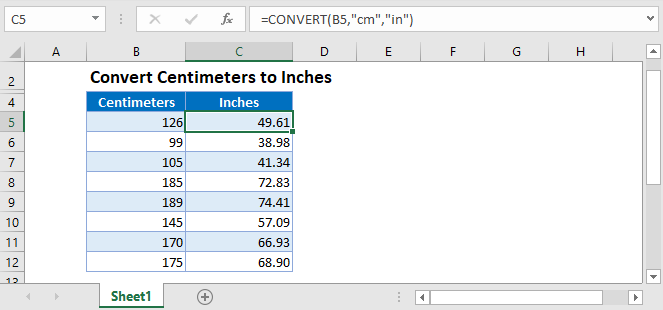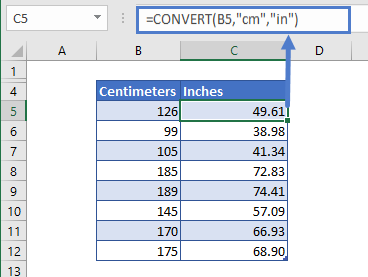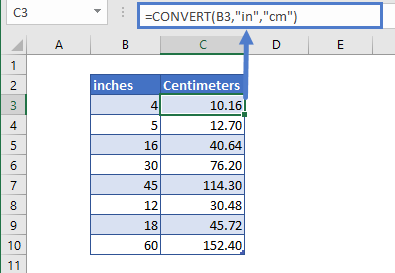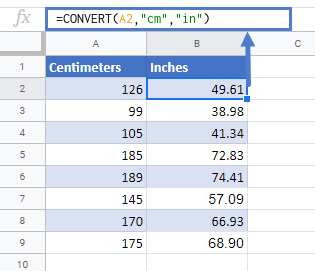# Convert Centimeters to Inches in Excel & Google Sheets

This tutorial will demonstrate how to convert centimeters to inches in Excel & Google Sheets.## CONVERT Function

The CONVERT function is the best method to convert centimeters to inches. Simply enter both the value and measurement types into the function.

``=CONVERT(B5,"cm","in")``Note: Enter the formula in cell C4 and drag it down.

## Convert Inches to Centimeters

By switching the measurements arguments, we can convert the values given in inches to centimeters:

``=CONVERT(B3,"in","cm")``## Convert Values in Centimeters to Inches in Google Sheets

The conversion of centimeters to inches using the CONVERT Function works the same in Google Sheets as in Excel:### Excel Practice Worksheet

Practice Excel functions and formulas with our 100% free practice worksheets!